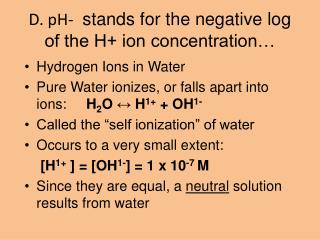# D. pH- stands for the negative log of the H+ ion concentration… - PowerPoint PPT PresentationDownload PresentationD. pH- stands for the negative log of the H+ ion concentration…

D. pH- stands for the negative log of the H+ ion concentration…Download Presentation## D. pH- stands for the negative log of the H+ ion concentration…

- - - - - - - - - - - - - - - - - - - - - - - - - - - E N D - - - - - - - - - - - - - - - - - - - - - - - - - - -
##### Presentation Transcript

1. D. pH- stands for the negative log of the H+ ion concentration… • Hydrogen Ions in Water • Pure Water ionizes, or falls apart into ions: H2O ↔ H1+ + OH1- • Called the “self ionization” of water • Occurs to a very small extent: [H1+ ] = [OH1-] = 1 x 10-7 M • Since they are equal, a neutral solution results from water

2. pH--All acids produce the hydrogen ion (H+) when placed in water. All bases produce the hydroxide ion (OH-) when placed in water.  • If something has a pH of 3 it means that the H+ ion concentration in 1 x 10-3 • Somebody (Sorensen) had a good idea to shorten it & make it a much easier system for most to use. Soren Sorensen (1868 - 1939)

3. pH scale pH scale : measures acidity/basicity ACID BASE 10x 100x 10x 10x 0 1 2 3 4 5 6 7 8 9 10 11 12 13 14 NEUTRAL Each step on pH scale represents a factor of 10. pH 5 vs. pH 6(10X more acidic) pH 3 vs. pH 5 (100X different) pH 8 vs. pH 13 (100,000X different)

4. Measuring pH • Why measure pH? • Solutions we use - everything from swimming pools, soil conditions for plants, medical diagnosis, soaps and shampoos, etc. • Sometimes we can use indicators, other times we might need a pH meter

5. Indicators are compounds whose colors are sensitive to pH – they change color based on pHSome of the many pH Indicators and their ranges

6. Measuring pH with wide-range paper 1. Moisten indicator strip with a few drops of solution, by using a stirring rod. 2.Compare the color to the chart on the vial – read the pH value.

7. Acid-Base Indicators • Although useful, there are limitations to indicators: • usually given for a certain temperature (25 oC), thus may change at different temperatures • what if the solution already has color, like paint? • the ability of the human eye to distinguish colors is limited

8. Acid-Base Indicators • A pH meter may give more definitive results • some are large, others portable • works by measuring the voltage between two electrodes; typically accurate to within 0.01 pH unit of the true pH • needs to be calibrated

9. Titration • Titration is the process of adding a known amount of solution of known concentration to determine the concentration of another solution • Remember? - a balanced equation is a mole ratio

10. Buffers • Buffers are solutions in which the pH remains relatively constant, even when small amounts of acid or base are added • made from a pair of chemicals: a weak acid and one of it’s salts; or a weak base and one of it’s salts

11. Buffers • A buffer system is better able to resist changes in pH than pure water • Since it is a pair of chemicals: • one chemical neutralizes any acid added, while the other chemical would neutralize any additional base • AND, they produce each other in the process!!!

12. So What??? • Buffers are useful! • They maintain the pH of human blood • In medicines they keep acidic meds from damaging our stomachs

13. VI. pH “power of hydrogen” • A. Water naturally ionizes into H3O+ & OH- making a very weak electrolyte  Ionization of water 2H2O H3O+ + OH- • B. Pure water will self ionize to produce a molar concentration ( M) of [H3O+] = 1 x 10-7 & [OH-] = 1 x 10-7 at 25oC (298K)

14. 1. using this info they can get an ionization constant for water Kw always = 1 x 10-14 Kw = [H3O+] x [OH-] • a. when one goes up the other goes down to reach equilibrium (Le Châtliers principle) • b. If you know the Kw (which you do) & you know one of the concentrations then you can calculate the other.

15. C. Since the pH #’s are so small they found another way to express them – they take the –log • 1. so pH= -log [H3O+] • 2. pH ranges from 0 to 14 • D. pOH deals with [OH-]-- pOH = -log[OH-] • E. pH + pOH = 14

16. IV. Titration • A. equations • 1. M1V1 = M2V2 • 2. Start with the balanced equation for the neutralization reaction. • 3. Determine the moles of acid/base from the known solution used during the titration. • 4. Determine the moles of solution of the unknown solution used during the titration. • 5. Determine the molarity of the unknown solution.

17. In a titration, 27.4 mL of 0.0154M Ba(OH)2 is added to a 20mL sample of HCl solution of unknown concentration. What is the molarity of the acid solution? • Ba(OH)2 + 2HCl = 2H2O + BaCl2 • .0154M Ba(OH)2 = mol = 4.15 x 10-4 mol Ba(OH)2 • .0274 L

18. 4.15 x 10-4 mol Ba(OH)2 2mol HCl = 1mol Ba(OH)2 • 8.316 x 10-4 mol HCl • molarity = mol = 8.316 x 10-4 mol HCl = .04M HCl L .020L HCl

19. OR M1V1 = M2V2